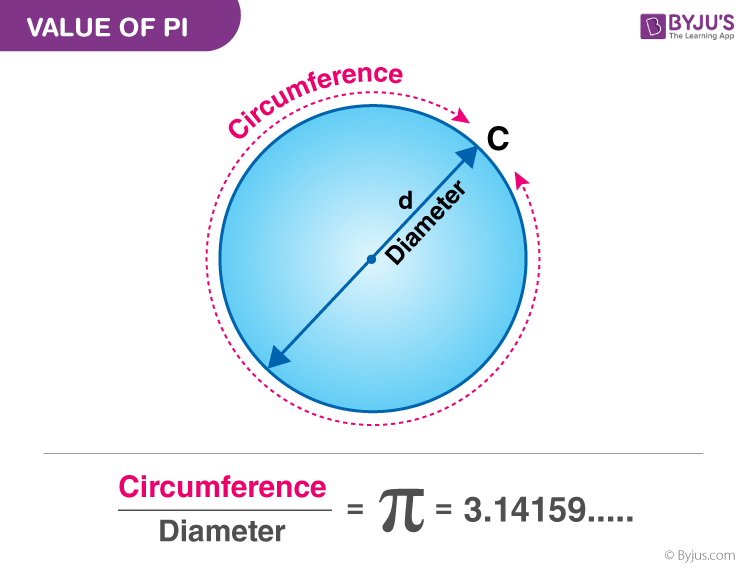# What is Pi?

Pi is a mathematical concept which represents the ratio between the circumference and the diameter of a circle. The pi value was first calculated by Archimedes of Syracuse. The symbol π was first popularized by Leonhard Euler in 1737.

### Value of pi

The pi is a ratio and is obtained from a circle. If the diameter and the circumference of a circle are known, the value of pi will be as π = Circumference/ Diameter.#### Value of Pi (π) in Decimal

The pi value up to the first 100 decimal places is:

3.14159 26535 89793 23846 26433 83279 50288 41971 69399 37510 58209 74944 59230 78164 06286 20899 86280 34825 34211 7067

#### Value of Pi (π) in Fractions

The pi value in fraction is 22/7. It is known that pi is an irrational number which means that the digits after the decimal point are never-ending and being a non-terminating value. Therefore, 22/7 is used for everyday calculations.

Check out the video given below to know more about pi(12)(3)